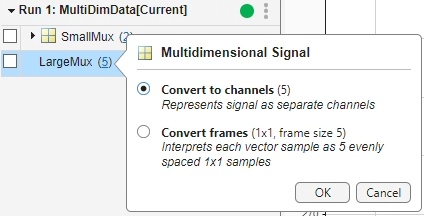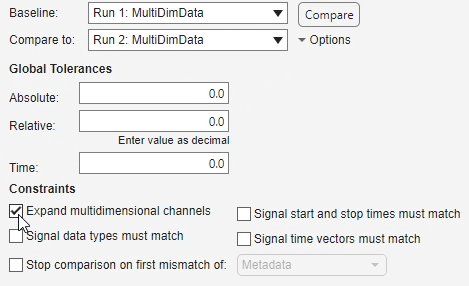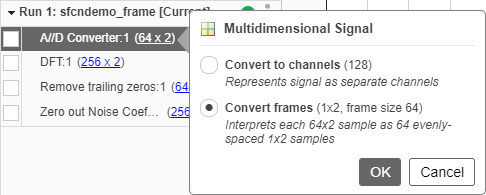Analyze Multidimensional Signal Data

When you log multidimensional signal data or import multidimensional data into the Simulation Data Inspector, you can choose to view and analyze the data as:

• A single signal with multidimensional sample values.

• A set of signals with scalar sample values: one signal, called a channel, for each element of the multidimensional data.

When the multidimensional data is frame-based, you can also use the Simulation Data Inspector to remove the buffering from each frame.

You can control how you view and analyze multidimensional signal data using the Record block or the Simulation Data Inspector. When you change the representation of a multidimensional signal in the Record block, the change is reflected in the Simulation Data Inspector and vice versa.

Convert Representation of Multidimensional Data

The way the Simulation Data Inspector initially represents a multidimensional signal depends on the number of elements in each sample of the signal. By default:

• Signals with samples that contain fewer than four elements are represented as channels.

• Signals with samples that contain more than four elements are represented as a single signal with multidimensional sample values.

• Variable-size signals are always represented as a single signal with multidimensional values.

When the default behavior for a signal with fixed dimensions does not provide the signals you need for your analysis, you can convert the representation from a single signal to channels or from channels to a single signal.

For example, consider this output from a model that creates two composite signals using Mux blocks:

• Large Mux contains five elements and is displayed as a single signal by default.

• Small Mux contains two elements and displayed as two channels by default.

The run includes one row for the Large Mux signal and three rows for the Small Mux signal. By default, the Simulation Data Inspector groups signals by data hierarchy, so one row groups the signals for each channel in the Small Mux signal, and each channel has a row.Signals with multidimensional data and the grouping rows for multidimensional signals represented as channels indicate the dimensions. To convert the representation of a signal with multidimensional data, you can click the dimensions and select an option from the menu. For example, to convert the Large Mux signal to channels, click the dimensions, 5, and select Convert to channels.To convert the Small Mux signal to a single signal, click the dimensions, 2, for the grouping row and select Convert to multidimensional signal.When you convert a signal from channels to a single signal, any plotted channels are cleared from the view because the signals for the channels no longer exist.

You can also convert the representation of multidimensional signals in the Simulation Data Inspector programmatically using the expand and collapse functions.

Plot Multidimensional Signal Data

Most visualizations in the Simulation Data Inspector, including time plots, XY plots, maps, and sparklines, only support plotting scalar signals. To plot data from a multidimensional signal on one of these visualizations, convert the signal to channels. You can use the conversion menus that you access by clicking the signal dimensions or the menu that appears on a subplot that does not support multidimensional data when you try to plot the signal.

For example, if you try to plot the Large Mux signal on a time plot, the menu gives you the option to change the active subplot to an array plot, which does support multidimensional data, or to convert the representation of the signal.To view multidimensional data, use the array plot in the Simulation Data Inspector. The array plot supports scalar and multidimensional signals, including variable-size signals. You can add an array plot to the subplot layout using the menu that appears when you try to plot a multidimensional signal on a plot that only supports scalar signals or using the Visualization Gallery. For more information about using the array plot, see View Multidimensional Signals Using the Array Plot. The Record block does not support the array plot.

Only time plots support plotting signals programmatically. You can plot channel data on a time plot programmatically. You cannot plot data for a signal with multidimensional sample values programmatically. Use the expand function to convert a single multidimensional signal to channels, which you can plot on a time plot programmatically using the plotOnSubPlot function.

Compare Multidimensional Signals

By default, when you compare multidimensional signals represented as a single signal or runs that contain multidimensional signals represented as a single signal, the Simulation Data Inspector converts the multidimensional signal to channels and computes a comparison result for each channel. The conversion happens for the comparison only and does not affect the way the signal is represented on the Inspect pane. The Simulation Data Inspector does not support comparing variable-size signals or signals with multidimensional sample values.

If you do not want to compute comparison results for the channels in multidimensional signals, you can configure comparisons to skip multidimensional signals instead of computing the channel results. To configure a comparison to skip multidimensional signals, on the Compare pane, click More, then clear Expand multidimensional channels.You can also configure whether programmatic comparisons compute results for multidimensional signals using the Simulink.sdi.compareRuns function with the ExpandChannels name-value argument.

Convert Representation of Frame-Based Data

Some applications buffer several samples of a signal into a frame to process with a single computation instead of processing each sample in the frame separately. When you work with frame-based signals, you can analyze the data frame by frame, or you can remove the buffering and analyze the signal without the frames.

You can remove frame buffering from signals logged to the Simulation Data Inspector using the menu that appears when you click the dimensions next to a multidimensional signal or the menu that appears when you try to plot multidimensional data on a plot that only supports scalar data.

For example, consider this run created by logging data for the analog to digital converter output in the model sfcndemo_frame. The model buffers the samples into a frame and performs a discrete Fourier transform on each frame. To remove the frame buffering in the Simulation Data Inspector, click the dimensions for the signal, 64×2, and select Convert frames. The conversion interprets each sample as 64 evenly spaced samples with dimensions 1-by-2. Because the unbuffered signal only contains two elements, conversion also displays the results as separate channels with scalar sample values.You can also convert the frames for a frame-based signal in the Simulation Data Inspector programmatically using the convertToFrames function. The Simulation Data Inspector does not support converting frames for imported data.

When you log frame-based data from a model, you can control how the data is logged by specifying the Input Processing setting for the logged signal in the model using the Instrumentation Properties dialog box or the Simulink.sdi.setSignalInputProcessingMode function. You can configure the input processing for the signal as frame-based or sample-based.

• Sample-based — The logged data retains the buffering, and each sample of the logged signal represents a frame. The Simulation Data Inspector interprets the data as a multidimensional signal, where each element of a sample represents a separate channel.

• Frame-based — Logging removes the buffering and treats each column in the samples as a separate channel.

When you log frame-based signals as sample-based, you can still convert the frames in the Simulation Data Inspector after simulation.